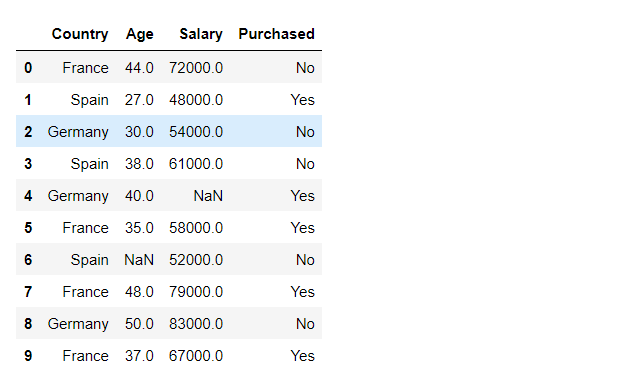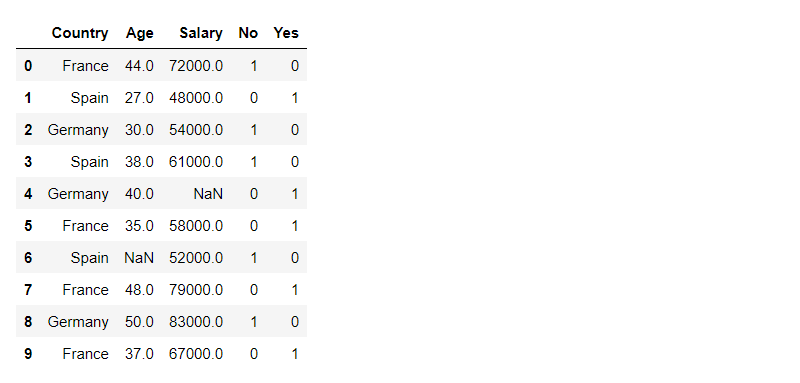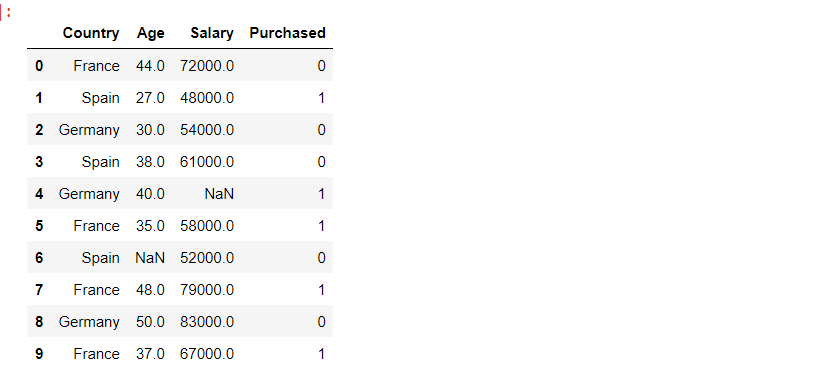GeeksforGeeks App
Open AppBrowser
Continue

# How to convert categorical string data into numeric in Python?

The datasets have both numerical and categorical features. Categorical features refer to string data types and can be easily understood by human beings. However, machines cannot interpret the categorical data directly. Therefore, the categorical data must be converted into numerical data for further processing.

There are many ways to convert categorical data into numerical data. Here in this article, we’ll be discussing the two most used methods namely :

• Dummy Variable Encoding
• Label Encoding

In both the Methods we are using the same data, the link to the dataset is here

## Method 1: Dummy Variable Encoding

We will be using pandas.get_dummies function to convert the categorical string data into numeric.

Syntax:

pandas.get_dummies(data, prefix=None, prefix_sep=’_’, dummy_na=False, columns=None, sparse=False, drop_first=False, dtype=None)

Parameters :

• data : Pandas Series, or DataFrame
• prefix : str, list of str, or dict of str, default None. String to append DataFrame column names
• prefix_sep : str, default ‘_’. If appending prefix, separator/delimiter to use.
• dummy_na : bool, default False. Add a column to indicate NaNs, if False NaNs are ignored.
• columns : list-like, default None. Column names in the DataFrame to be encoded.
• sparse : bool, default False. Whether the dummy-encoded columns should be backed by a SparseArray (True) or a regular NumPy array (False).
• drop_first : bool, default False. Whether to get k-1 dummies out of k categorical levels by removing the first level.
• dtype : dtype, default np.uint8. It specifies the data type for new columns.

Returns : DataFrame

## Python3

 `# importing pandas as pd``import` `pandas as pd`

## Python3

 `# importing data using .read_csv() function``df ``=` `pd.read_csv(``'data.csv'``)` `# printing DataFrame``df`

Output:### Step 3: Converting Categorical Data Columns to Numerical.

We will convert the column ‘Purchased’ from categorical to numerical data type.

## Python3

 `# using .get_dummies function to convert``# the categorical datatype to numerical``# and storing the returned dataFrame``# in a new variable df1``df1 ``=` `pd.get_dummies(df[``'Purchased'``])` `# using pd.concat to concatenate the dataframes``# df and df1 and storing the concatenated``# dataFrame in df.``df ``=` `pd.concat([df, df1], axis``=``1``).reindex(df.index)` `# removing the column 'Purchased' from df``# as it is of no use now.``df.drop(``'Purchased'``, axis``=``1``, inplace``=``True``)` `# printing df``df`

Output:## Method 2:  Label Encoding

We will be using .LabelEncoder() from sklearn library to convert categorical data to numerical data. We will use function fit_transform() in the process.

Syntax :

fit_transform(y)

Parameters :

• y : array-like of shape (n_samples). Target Values.

Returns : array-like of shape (n_samples) .Encoded labels.

## Python3

 `# importing pandas as pd``import` `pandas as pd`

## Python3

 `#importing data using .read_csv() function``df ``=` `pd.read_csv(``'data.csv'``)` `#printing DataFrame``df`

Output:### Step 3 : Converting Categorical Data Columns to Numerical.

We will convert the column ‘Purchased’ from categorical to numerical data type.

## Python3

 `# Importing LabelEncoder from Sklearn``# library from preprocessing Module.``from` `sklearn.preprocessing ``import` `LabelEncoder` `# Creating a instance of label Encoder.``le ``=` `LabelEncoder()` `# Using .fit_transform function to fit label``# encoder and return encoded label``label ``=` `le.fit_transform(df[``'Purchased'``])` `# printing label``label`

Output:

`array([0, 1, 0, 0, 1, 1, 0, 1, 0, 1])`

Time Complexity:  O(n log n) to O(n^2) because it involves sorting and finding unique values in the input data. Here, n is the number of elements in the df[‘Purchased’] array.

Auxiliary Space: O(k)  where k is the number of unique labels in the df[‘Purchased’] array.

## Python3

 `# removing the column 'Purchased' from df``# as it is of no use now.``df.drop(``"Purchased"``, axis``=``1``, inplace``=``True``)` `# Appending the array to our dataFrame``# with column name 'Purchased'``df[``"Purchased"``] ``=` `label` `# printing Dataframe``df`

Output:My Personal Notes arrow_drop_up